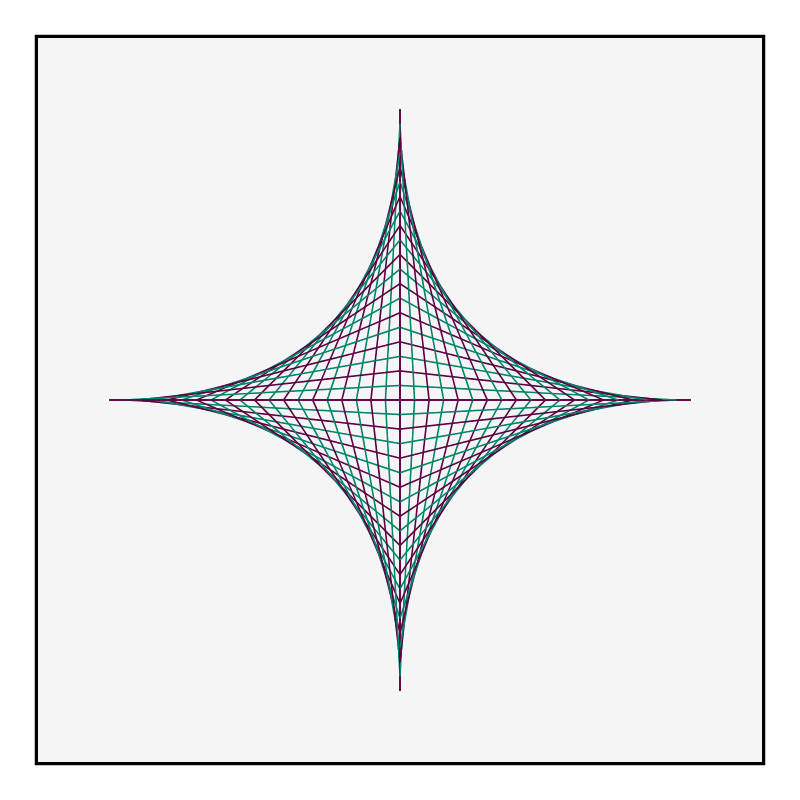Star construction using straight lines.Author: Brent Yorgey
> import Diagrams.Backend.SVG.CmdLine
> {-# LANGUAGE NoMonomorphismRestriction, TupleSections #-}
> import Diagrams.Prelude hiding (connect)
> import Data.Colour.SRGB (sRGB24read)
> colors = map sRGB24read ["#5E0042", "#00856A"]

To create one quarter of the diagram, we connect the corresponding points with headless arrows and alternate the line colors.

> quarter n = mconcat [arrowBetween'
>         & shaftStyle %~ lw thin . lc (colors !! ((xCoord1 p) mod 2)))
>   (fst p) (snd p) | p <- ps]
>   where
>     xCoord1 = round . fst . unp2 . fst
>     ps = zip xs (reverse ys)
>     (xs, ys) = pts n

The final diagram is created by assembling four copies of the above.

> d n = half === rotateBy (1/2) half
>   where
>     half = (rotateBy (1/4) q ||| q) # centerX
>     q = quarter n
> pts n = (map (p2 . (,0)) [0..n], map (p2 . (0,)) [0..n])
> example = pad 1.1 \$ d 20 # centerXY atop square 50 # fc whitesmoke
> main = mainWith (example :: Diagram B)